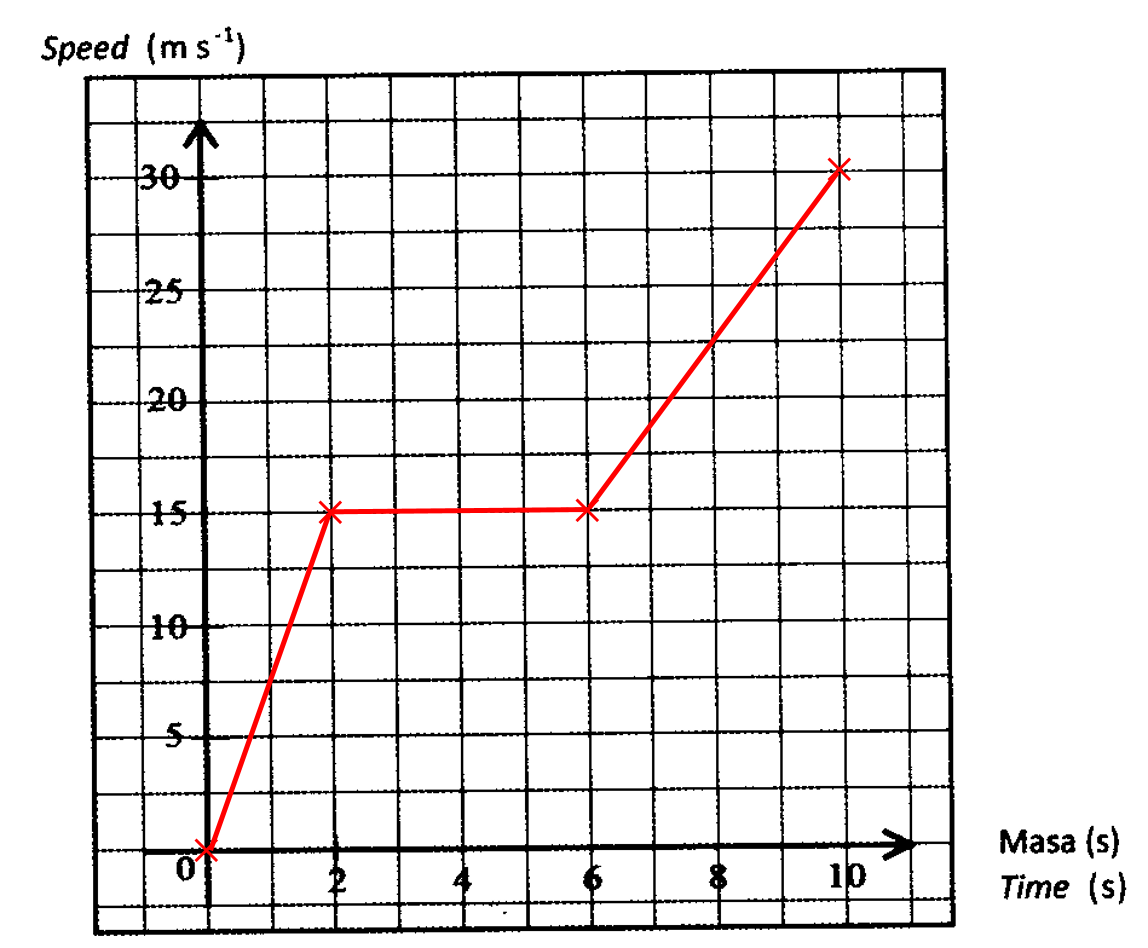# SPM Mathematics Trial 2021 (Kelantan), Paper 2 (Question 13)

Question 13:
Table 2 shows the speed and time of a particle in 10 seconds.

 Speed (ms-1) 0 15 15 30 Time (s) 0 2 6 10
(a) Based on Table 2, draw the speed-time graph in figure in the answer space. [2 marks]

(b) Based on the graph drawn in Figure in the answer space, state:
(i) Uniform speed, in ms-1, of the particle. [1 mark]
(ii) The duration of time, in second, the particle moves in uniform speed. [1 mark]

(c) Calculate the rate of change of speed, in ms-2, for the last 4 seconds. [2 marks]

(d) Calculate the average speed, in ms-1 of the particle in period of 10 seconds. [3 marks]

(a)Solution:
(a)(b)(i)
Uniform speed of the particle is 15 ms-1.

(b)(ii)
Duration of time the particle moves in uniform speed = 6 – 2 = 4 seconds

(c)

(d)